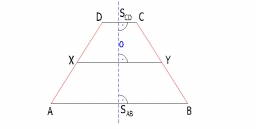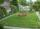# Garden G

The rectangular, trapezoidal garden has a base length of 81m, 76m, and a vertical arm of 12m. Calculate how many m2 of the area will remain for planting greenery if 1/3 of the area is built. Calculate the consumption of mesh for land fencing.

S =  1256 m2
o =  106 m

### Step-by-step explanation:Did you find an error or inaccuracy? Feel free to write us. Thank you!Tips to related online calculators
Need help to calculate sum, simplify or multiply fractions? Try our fraction calculator.
Pythagorean theorem is the base for the right triangle calculator.

#### You need to know the following knowledge to solve this word math problem:

We encourage you to watch this tutorial video on this math problem:

## Related math problems and questions:

• Garden exchangeThe garden has a rectangular trapezoid shape, the bases of which have dimensions of 60 m and 30 m and a vertical arm of 40 m. The owner exchanged this garden for a parallelogram, which is 7/9 of the area of a trapezoidal garden. What is the size of the ne
• Trapezoid RTThe plot has a shape of a rectangular trapezium ABCD, where ABIICD with a right angle at the vertex B. side AB has a length 36 m. The lengths of the sides AB and BC are in the ratio 12:7. Lengths of the sides AB and CD are a ratio 3:2. Calculate consumpti
• GardenThe area of a square garden is 2/9 of triangle garden with sides 160 m, 100 m, and 100 m. How many meters of fencing need to fence a square garden?
• The gardenThe garden has the shape of a rectangular trapezium. The bases have lengths of 27 meters and 36 meters, the trapezoid's height is 12 meters. Calculate how much fence will cost this garden if one meter costs 1.5 €?
• Rectangular gardenRectangular garden has a length of 48.7 m, a width of 6.3 meters shorter than the length. How much mesh should be bought for its fencing if the gate is 2.9 m long and the gate 1.1 m? What is the area of the garden?
• Trapezoidal prismCalculate the surface of the quadrilateral prism ABCDA'B'C'D 'with the trapezoidal base ABCD. The height of the prism is 12 cm; ABCD trapezoidal data: AB base length is 8 cm, CD base length is 3 cm, BC arm length is 4 cm, and AC diagonal length is 7 cm. L
• Land - isosceles trapezoidCalculate the building plot's content and perimeter in the form of an isosceles trapezoid with bases 120m, 95m, and height 50m.
• Rectangular trapezoidThe rectangular trapezoid ABCD is: /AB/ = /BC/ = /AC/. The length of the median is 6 cm. Calculate the circumference and area of a trapezoid.
• ISO Triangle V2The perimeter of RR triangle (isosceles) is 474 m, and the base is 48 m longer than the arms. Calculate the area of this triangle.
• Land boundaryThe land has the shape of a right triangle. The hypotenuse has a length of 30m. The circumference of the land is 72 meters. What is the length of the remaining sides of the land boundary?
• Garden fenceThe garden has the shape of a rectangular triangle with an area of 96 square meters and a 16 m long one leg. How many meters of the fence need to be fenced?
• LandRectangular triangular land has area 30 square meters and 12 meters long leg. How many meters of the fence do you need for fencing this land?
• Rectangular gardenThe sides of the rectangular garden are in ratio 1: 2. The diagonal has a length of 20 meters. Calculate the area and perimeter of the garden.
• Trapezoid MOThe rectangular trapezoid ABCD with the right angle at point B, |AC| = 12, |CD| = 8, diagonals are perpendicular to each other. Calculate the perimeter and area of ​​the trapezoid.
• GardenThe rectangular garden has a length 99 m and width 84 m. Calculate how many m2 will decrease its area if land by the ornamental fence with width 30 cm.
• GardensThe area of the square garden is 3/4 of the area of the triangular garden with sides of 80 m, 50 m, 50 m. How many meters of the fence do we need to fence a square garden?
• Rectangular trapeziumCalculate the perimeter of a rectangular trapezium when its content area is 576 cm2 and sice a (base) is 30 cm, height 24 cm.